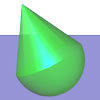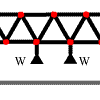#### You may also like### Lunar Leaper

Gravity on the Moon is about 1/6th that on the Earth. A pole-vaulter 2 metres tall can clear a 5 metres pole on the Earth. How high a pole could he clear on the Moon?### Wobbler

A cone is glued to a hemisphere. When you place it on a table in what position does it come to rest?### More Bridge Building

Which parts of these framework bridges are in tension and which parts are in compression?

# Bridge Builder

### Why use this problem

A great deal of insight into Latticework problems can be achieved without the need for any numerical computation at all: we rely on vector methods and the simple principles of tension and force to determine the character of the tensions and compressions in the bridge. The use of ropes and springs will make students think about the difference between an internal tension and an internal compression.

This problem will help students to realise that numerical and algebraic manipulation are not always necessary as the first step in a mechanics problem; it also helps to train students' self checking mechanisms.

This problem also reinforces basic ideas concerning vectors and components.

### Possible Approach

Students will probably be tempted to try to calculate the magnitude of all of the forces algebraically but that is unnecessary in this question. To help reinforce this idea, you could discuss the problem as a group, beginning with the question of how vectors will help us in the problem.

Although students should be encouraged to solve the problem without algebra, they may wish to perform an algebraic calculation to convince themselves that their deductions are indeed correct. Hopefully after some thought the principle will become clear and 'obvious' to the students.

The approach used in this question might seem unusual to many students and could form the basis for a discussion about mathematics. Although the question will not require any numerical calculation, a good answer will need to be clearly and accurately explained. Students could explain their answers to the group. Does the group feel that the answer is robust? Are there any weaknesses in the argument that might need clarification? Conversely, are there any particularly clear aspects of the argument? How do students feel about not using numbers? Are they aware that the lack of algebra does not in any way reduce the mathematical importance or significance of a task? Would an algebraic calculation involving the mass of the weight make the result any more believable?

### Key Questions

• What are the differences between a spring and a string?
• In order for a pin joint to experience no net forces what must be satisfied?
• How can vectors help us in this problem?

### Possible Extension

Once the concept of resolution of vector components is understood, there are several avenues of further investigation for this problem, such as the following
• The bridge in this question is made from 7 triangular segments. What happens if the weight is hanging from the centre of a bridge with more triangular segments?
• What happens if the bridge is made from isosceles-triangular segments instead of equilateral-triangular segments?
• What would happen if an extra row of triangles were jointed onto the top of the bridge?
• Which springs/ropes would experience the most/least internal forces?

In addition, there is a follow-up extension problem to this task: more bridge building .

### Possible Support

You could first try a simpler version of the problem in which the bridge is made from three triangular segments. What direction must the forces be pointing in the central two portions of the bridge?